# SSC CGL EXAMS 2018 | Reasoning Ability Practice Questions (Day-5)

Dear Aspirants, Here we have given the Important SSC Exam 2018 Practice Test Papers. Candidates those who are preparing for SSC 2018 can practice these questions to get more confidence to Crack SSC 2018 Examination.

[WpProQuiz 4098]

1) Select the related word from the given alternatives:

Amount of substance: Mole :: Weight : ?

a) Scale

b) Kilogram

c) Commodity

d) Measurement

2) What is the number of triangles in the given figure?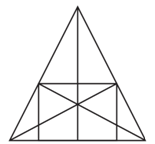a) 20

b) 24

c) 30

d) 36

3) A dice is numbered from 1 to 6 in different ways. If 1 is adjacent to 2, 4 and 6, then which of the following statements is necessarily true?

a) 3 is adjacent to 5

b) 1 is adjacent to 3

c) 3 is opposite to 5

d) 2 is opposite to 6

4) A picture is copied onto a sheet of paper 7.5 inches by 9.0 inches. A 1.0 inch margin is left all around. What area in square inches does the picture cover?

a) 45

b) 38.5

c) 40.5

d) 37.5

5) If South-East becomes North, North-East becomes West and so on. What will West become?

a) North – East

b) North – West

c) South – East

d) South – West

6) If you write down all the numbers from 1 to 100, then how many times do you write 3 ?

a) 18

b) 19

c) 20

d) 22

7) Sita introduced Mohan as the son of’ the daughter of the father of her uncle. Mohan is Sita’s

a) Brother

b) Father

c) Uncle

d) Grandfather

8) There are how many 4’s proceeded by 7 but not followed by 3?

5  9  3  2  1  7  4  2  6  9  7  4  6  1  3  2  8  7  4  1  3  8  3  2  5  6  7  4  3  9  5  8  2  0  1  8  7  4  6  3

a) Three

b) Four

c) Five

d) None

9) A Matrix is given below. The characters in matrix follow a certain trend, row-wise or column wise. Find out this trend and choose the missing character accordingly.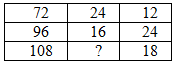a) 12

b) 16

c) 18

d) 24

10) In a class of 60 students where girls are twice that of boys, Preeti ranked 27th from the top. If there are 9 boys ahead of Preeti, how many girls are after her rank?

a) 24

b) 23

c) 22

d) 21

11) Identify the relationship that best represents the relationship among classes given below.

Teachers, women, clerks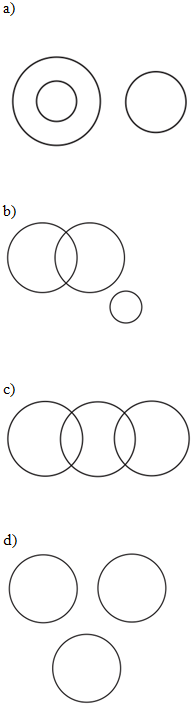12.

If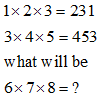a) 786

b) 867

c) 687

d) 678

13) Find the odd one

a) Flower : Petal

b) Cover : Page

c) Circle : Arc

d) Chair : leg

14) SENIPAH is to 9386715, and then HAPPINESS is To?

a) 577168399

b) 517668399

c) 517768399

d) 517763399

15. What value replace “?” in the below figure?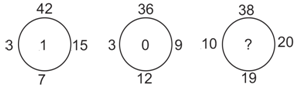a) 4

b) 0

c) 2

d) 1

It is unit of measurement.

Number of triangles is give below the figure.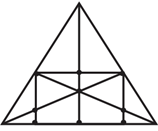The simplest triangles are ADE, AEF, DEK, EFK, DJK, FLK, DJB, FLC, BJG and LIC i.e. 10 in number.

The triangles composed of two components each are ADF, AFK, DFK, ADK, DKB, FCK, BKH, KHC, DGB and FIC i.e. 10 in number.

The triangles composed of three components each are DFJ and DFL i.e. 2 in number.

The triangles composed of four components each are ABK, ACK, BFI, CDG, DFB, DFC and BKC i.e. 7 in number.

The triangles composed of six components each are ABH, ACH, ABF, ACD, BFC and CDB i.e. 6 in number.

There is only one triangle i.e. ABC composed of twelve components.

There are 10 + 10 + 2 + 7 + 6+ 1 = 36 triangles in the figure.

Draw out the diagram and you will see that the width of the picture becomes 7.5 – 2 = 5.5 inch

The length becomes 9 – 2 = 7 inch. The area is now 5.5 x 7 = 38.5

South-East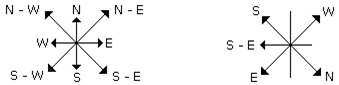Clearly, From 1 to 100, there are ten numbers with 3 as the unit’s digit – 3, 13, 23, 33, 43, 53, 63, 73, 83, 93 and ten numbers with 3 as the ten’s digit – 30, 31, 32, 33, 34, 35, 36, 37, 38, 39.

So, required number = 10 + 10 = 20.

Daughter of uncle’s father — Uncle’s sister — Mother; Mother’s son — Brother

7  4  2

7  4  6

7  4  1

7  4  6

Only at these places 4 is preceded by 7 but not followed by 3

In the first row, 72 ÷ (24/4) = 72 ÷ 6 = 12.
In the second row, 96 ÷ (16/4) = 96 ÷ 4 = 24.
Let the missing number in the third row be y.
Then, 108 ÷ (y/4) = 18 or  y/4 = 108/18 = 6 or  y = 24.

Given number of girls is twice that of boys.

–> G = 2B and G+B = 60

Therefore, G=40 and B= 20

Given Preeti ranked 27th from the top and 9 boys are ahead of Preeti.

Therefore, There are 17 girls ahead of Preeti.

Therefore, Number of girls who ranked after Preeti = 40 – (17+1) = 22.

Few teachers may be women few clerks also may be women.

Teachers cannot be clerks

Middle No. comes in 1st

Last No. comes in 2nd

First No. comes in 3rd.

In all other pairs, second is a part of the first.

S – 9

E – 3

N – 8

I – 6

P – 7

A – 1

H – 5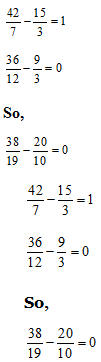*********************************

### SSC Reasoning Ability Practice Questions – (Day-2)